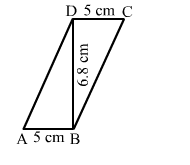# In the given figure, ABCD is a || gm in which AB = CD = 5 cm and BD ⊥ DC such that BD = 6.8 cm.

Question:

In the given figure, ABCD is a || gm in which AB = CD = 5 cm and BD ⊥ DC such that BD = 6.8 cm. Then, the area of || gm ABCD = ?

(a) $17 \mathrm{~cm}^{2}$

(b) $25 \mathrm{~cm}^{2}$

(c) $34 \mathrm{~cm}^{2}$

(d) $68 \mathrm{~cm}^{2}$Solution:

(c) 34 cm2

ar(parallelogram ABCD) = base × height = 5 ​× 6.8 =  34 cm2